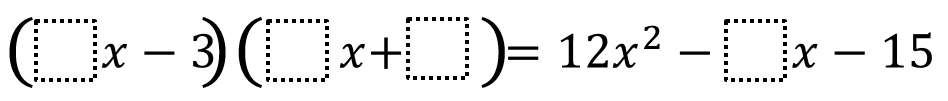Home > High School: Algebra > Multiplying Binomials

# Multiplying Binomials

Directions: Fill in the boxes with any numbers that make the equation true.### Hint

What number is the easiest to fill out? What are the factors of 12?

### Answer

There are many possibilities. Here are some: 2, 6, 5, and 8; 1, 12, 5, and 31

Source: Dane Ehlert

## Writing Equivalent Polynomial Expressions

Directions: Use the digits 1-9, at most one time each, to create a true statement. …